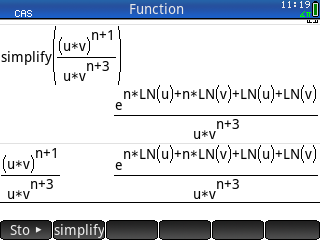# HP Forums

Full Version: Simplifying Rational Expressions
You're currently viewing a stripped down version of our content. View the full version with proper formatting.
How can I simplify the expression below with the HP Prime? I like to see u^n over v^2 as the result.If I make
simplify((u^(n+1)*v^(n+1))/(u*v^(n+3)))
I get a little bit further to u^(n+1)/(u*v^2), but it is not able to reduce the fraction to u^n/v^2. If I try to simplify the exptression u^(n+1)/(u*v^2) I get what you already got.

BTW: If I try expand(((u*v)^(n+1))/(u*v^(n+3))) with the TI89 Titanium I get (u*v)^n/v^(n+2).
If I do expand((u^(n+1)*v^(n+1))/(u*v^(n+3))) I get u^n/v^2.
So this is not perfect but better than the Prime.

Result with TI nspire CX II-T CAS:
expand(((u*v)^(n+1))/(u*v^(n+3))) --> (u*v)^n/(u*v^(n+3))
expand((u^(n+1)*v^(n+1))/(u*v^(n+3))) --> u^n/v^2

So it seams that the brackets in the numerator make things more difficult.

It's really strange because it is mathematically so simple.

Best

Raimund
Try to use, in sequence, "texpand" and then "simplify"

Greetings, Robmio
(07-08-2021 09:38 AM)robmio Wrote: [ -> ]Try to use, in sequence, "texpand" and then "simplify"

Greetings, Robmio

That's what I get:

[attachment=9625]
Hi, you don't have to use "expand", but "texpand", followed by "simplify".
CAS> simplify(pow2exp((u*v)^(n+1)/(u*v^(n+3)))) ﻿ ﻿ ﻿ ﻿ ﻿ ﻿ ﻿ ﻿ ﻿ ﻿ ﻿ ﻿ ﻿ ﻿ → u^n/v^2
CAS> simplify(pow2exp(u^(n+1)*v^(n+1)/(u*v^(n+3)))) ﻿ ﻿ ﻿ ﻿ ﻿ ﻿ → u^n/v^2
(07-08-2021 10:26 AM)robmio Wrote: [ -> ]Hi, you don't have to use "expand", but "texpand", followed by "simplify".

Thanks! Amazing, this works:
[attachment=9627]
(07-08-2021 11:59 AM)Albert Chan Wrote: [ -> ]CAS> simplify(pow2exp((u*v)^(n+1)/(u*v^(n+3)))) ﻿ ﻿ ﻿ ﻿ ﻿ ﻿ ﻿ ﻿ ﻿ ﻿ ﻿ ﻿ ﻿ ﻿ → u^n/v^2
CAS> simplify(pow2exp(u^(n+1)*v^(n+1)/(u*v^(n+3)))) ﻿ ﻿ ﻿ ﻿ ﻿ ﻿ → u^n/v^2

This works great. Thanks!

[attachment=9628]
My afx-2.0 calculator outputs:
simplify ((U×V)^(N+1)/((U×V)^(N+3)))
result is 1/(U^2×V^2)
Is this correct?
EDIT
My input mistake:
(u×v)^(n+1)/(u×v^(n+3))
Yes, Prime wins.
(07-09-2021 03:11 PM)Hlib Wrote: [ -> ]My afx-2.0 calculator outputs:
simplify ((U×V)^(N+1)/((U×V)^(N+3)))
result is 1/(U^2×V^2)
Is this correct?

The original expression is ((U*V)^(N+1))/(U*(V^(N+3)), my algebra FX 2.0 isn't able to simplify it.
Yes, I have edit my mistake.
Reference URL's
• HP Forums: https://www.hpmuseum.org/forum/index.php
• :